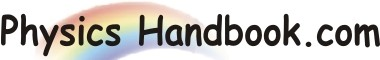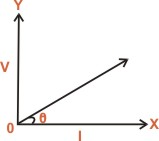HOME TOPICS DEFINITIONS TABLES LAWS INVENTIONS EXPERIMENTS QUIZ VIDEOS
 Ohm's Law The relationship between voltage across and current through a conductor was first discovered by German Scientist George Simon Ohm. This relationship is called Ohm's law. It states that the current (I) flowing through a conductor is directly proportional to the potential difference (V) across its ends provided the physical conditions do not change. i.e   I ∞ V    or    V / I = Constant = R Where R is a constant of proportionality and is called resistance of conductor. The unit of resistance is ohm. If V is measured in volts and I in amperes, R is given in ohms. For example, if the potential difference between points A and B of the conductor is V and current flowing is I, then V / I will be constant and equal to R, resistance of the conductor between points A and B. If V is doubled up, current will also be double up so that ratio V / I is constant. If a graph is drawn between applied potential difference (V) and current (I) flowing through the conductor, it will be a straight line passing through the origin. The slope of the graph gives the resistance of the conductor ( tanθ = V / I = R ).Those conductors which obey ohm's law are called ohmic conductors. The ohm's law is true only for ohmic conductors. If material is not ohmic, relation between V and I will not be linear so that R is not constant.